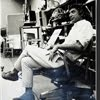## Eugene Ray SDSU 1970'sEugene Ray 1970's. photo credit: Tom Davis

## Tuesday, September 30, 2014

### APOCALYPSE @ SDSU / silver ship biotronic concepts

+FLASH OF LIGHT IN+
>(sdsu /  1969-1996<
>our years of effort)<
+THE EVENING SKY+
^^^^^^^^^^^^^^
>(if you like synchronicity, our silver ship<
+(SILVER SHIP STUDIO+
+/>1699 NAUTILUS ST.)+
>address in lajolla was 1699 nautilus street)<
^^^^^^^^^^^^^^^^^^^^^^^^^^^
+THE NAUTILUS SHELL HAS+
+TO BE OUR PROFOUNDEST+
>(biomorphic structure<
>at its very strongest)<
+LESSON FOR STRUCTURES+
^^^^^^^^^^^^^^^^^^^^^
>(our exhibitions of 1995 and 1975<
>shown in tonight's photo lexicon)<
^^^^^^^^^^^^^^^^^^^^^
>it was my pleasure to design one of our<
>last sdsu exhibitions using econo logs<
+(SDSU / ED 1995)+
>waste plywood cores bought for \$5<
>(commonly known as peeler logs)<
^^^^^^^^^^^^^^^^^^^^^^^^^
>we used these to hang our house models<
>exhibited on circular composite wood<
+(SDSU / ED 1995)+
>chip precut rounds 16 inch diameter<
^^^^^^^^^^^^^^^^^^^^^^^^^
>in the early 1990s our design research was<
>successful in all california competitions &<
+FRANK LLOYD WRIGHT)+
>this exhibition was one of our very best<
>(my taliesin west lecture @ this time)<
^^^^^^^^^^^^^^^^^^^^^^^^^^^^>in the mid 1970s our work w/ pneumatic<
>structures was strong & dave fobes air<
+(SDSU / ED 1975)+
>brush renderings are seen featured<
^^^^^^^^^^^^^^^^^^^^^^^^^
>in the mid 1970s i installed a comprehensive<
>exhibition of our advanced structures with<
+(SDSU / ED 1975)+
>light weight tensile & biomorphics special<
>w/ biotronic struct. domes, rammed earth<
^^^^^^^^^^^^^^^^^^^^^^^^^^^^
>i wrote titled "environ media"…collage was<
+(SDSU / ED 1975)+
>a technique i had started in new orleans &<
>perfected in calif w/ video/slide lectures<
^^^^^^^^^^^^^^^^^^^^^^^^^^^
>my goal was teaching advanced design<
>concepts expressed in graphics that had<
+(SDSU / ED 1975)+
^^^^^^^^^^^^^^^^^^^^^^
>in the mid 1970s our research & development<
>had reached a vital plateau seen in this solar<
+(SDSU / ED 1975)+
>heated residential prototype exhibit above<
^^^^^^^^^^^^^^^^^^^^^^^^^^^^^^
>this vignette in our sdsu studio classroom 301<
+(SDSU / ED 1973)+
>angeles who sent a crew to document<
>my work w/ radiant color and light<
^^^^^^^^^^^^^^^^^^^^^^^
>so the cbs television station in los angeles<
>sent a crew down to document our work<
+BIO- INVENTIONS '73)+
>but they brought lights that ruined our<
^^^^^^^^^^^^^^^^^^^^^^^^
>grad student kotaro nakamura aided me<
>in a regional shopping center concept<
+/ "DESERT INCIDENTS")+
>that i evolved into "desert incidents"<
>this shall be the main design i leave<
^^^^^^^^^^^^^^^^^^^^^^
>in new orleans i had invented a technique<
>collaging fluorescent color paper on the<
>design graphics to express radiance as<
+PHOTO TECHNIQUE)+
>photographed w/ ulta violet light and<
>using infra-red film in my leica 3-f<
^^^^^^^^^^^^^^^^^^^^^^^^^
>i am very grateful to jim bourke who took<
>this photo & alice anda my last student<
>assistant who aided me when i retired<
+(GRATITUDE : MY STUDENT+
+ASSIST'S THRU THE YEARS)+
>after contracting malaria in brazil after<
>my last international exhibition/lecture<
^^^^^^^^^^^^^^^^^^^^^^^^^^

+"DESERT INCIDENTS" & DJED DEVICE+
+OFFERED IN CA. PALM DESERT AREA+
>(ufo/et back engineered technology<
+TO PROVIDE OUR DESIGN CONCEPTS+
^^^^^^^^^^^^^^^^^^^^^^^^^^^^^^^^
eugene ray, mfa, architect
professor emeritus, sdsu